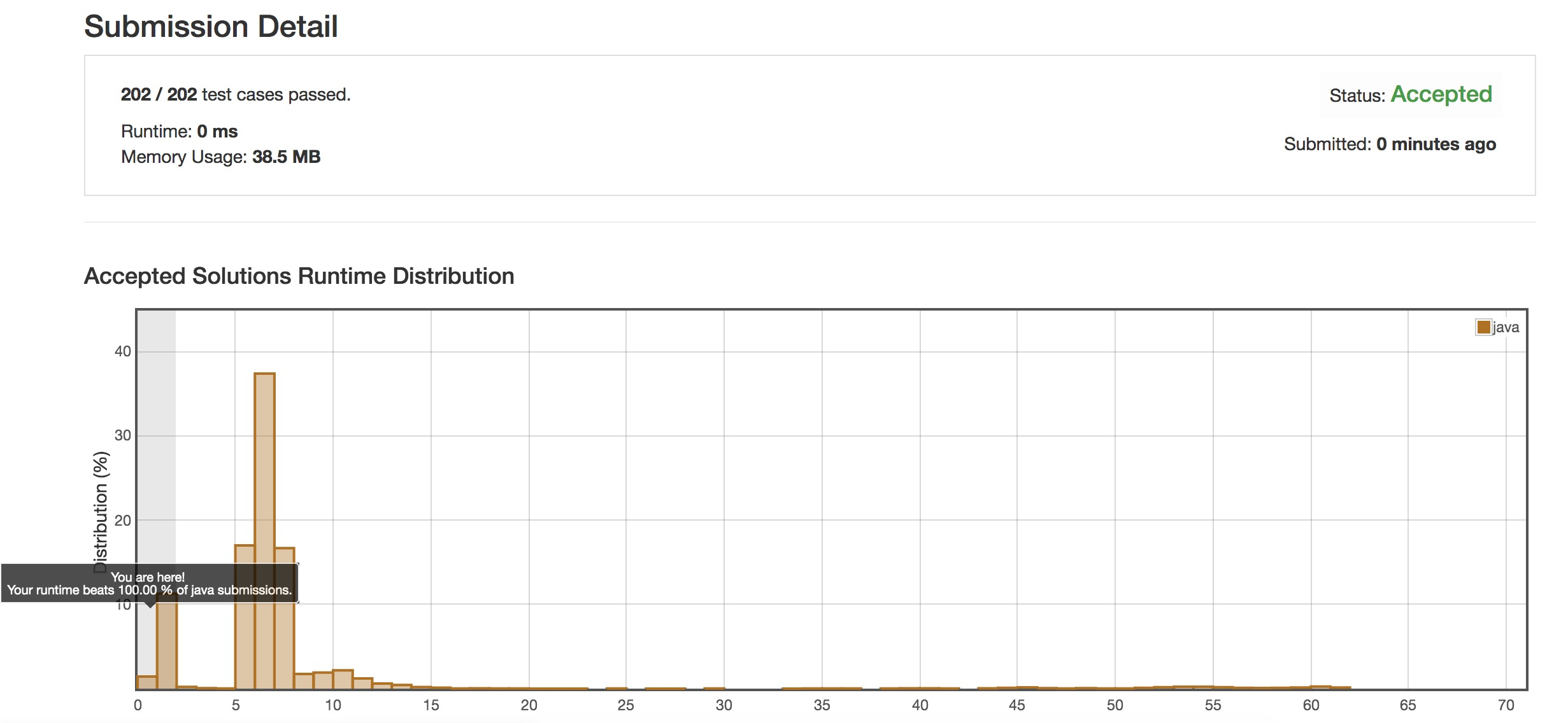2019/04/09 05:34

# 题目

输入: [-2,1,-3,4,-1,2,1,-5,4],



# 分析

## 初步分析

f(n)为前n个元素的最大子序和的值

• 当最大子序包含nums[n]，则f(n+1)的求解如下：

如果nums[n+1]>0 则f(n+1)=f(n)+nums[n+1]
如果nums[n+1]<0 则f(n+1)=f(n)

• 当最大子序不包含nums[n]，则f(n+1)的求解如下：

来一个nums[n+1]只会对以nums[n]结尾的每个子序的最大和产生更新影响，同时如果前面的子序和都是负的话，则nums[n+1]则是最大子序和，所以只需要计算出这部分更新后的最大子序和与f(n)对比求最大值即为f(n+1)的最大子序和

即f(n+1)=max(f(n),以第n个元素结尾的最大子序和+nums[n+1],nums[n+1])


g(n)为以第n个元素结尾的最大子序和，则g(n+1)的解如下：

当g(n)>0 则g(n+1)=g(n)+nums[n+1]



## 转换思路

假如我知道了每个g(n)的值，那么f(n)其实就是max(g(k)) 0<=k<=n


## 套路总结

• 很多时候动态规划的出题者不会给你一个一眼就能看出的状态，这时候就可能需要重新去找一个等价命题的状态，比如对于该题，你能够快速想到g(n)这个状态
• 假如想不到对应的等价命题的状态，看能否根据已有的简单的动态规划的解来求解问题，比如我已知了g(n)，能够利用g(n)作为突破口来推导目标问题的答案
• 某个子序的解可能是所有以某个元素结尾的子序的解的max或者min等其他函数，以某个元素结尾的子序的解可以简化好多问题

# 代码

public static int maxSubArray(int[] nums) {
int fn = nums;
int lastGn = nums;
for (int i = 1; i < nums.length; i++) {
if (lastGn > 0) {
lastGn += nums[i];
} else {
lastGn = nums[i];
}
if (lastGn > fn) {
fn = lastGn;
}
}
return fn;
}


# 跑分### 作者的其它热门文章

0
4 收藏

1 评论
4 收藏
0